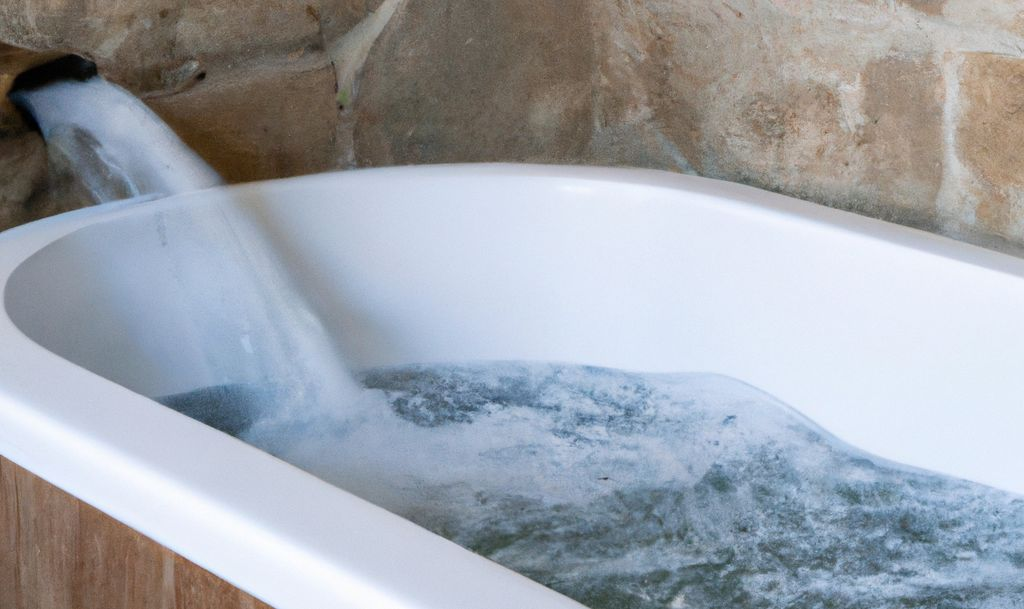# How to answer Rate of Flow questions (GCSE Maths)

Rate of flow is a topic that’s sometimes omitted in GCSE Maths text books, or perhaps included in the form of just a question or two in the section on compound measures. It’s only needed for the Higher Tier so you don’t need to worry about it if you’re doing Foundation.

## What is rate of flow?

Rate of flow is the speed at which something moves into or out of a container, or past a particular point (e.g. water in a river). It’s used for a fluid or a substance that flows in a similar manner, for example water draining out of a bathtub or grain being poured into a silo.

It’s measured as [a unit of volume] / [a unit of time],
for example cm³ per second, litres per minute, m³ per hour.

You often need to be able to convert between units in order to complete calculations involving rate of flow.

For volume you’ll need these equivalents:
1 cm³ = 1 ml so 1000 cm³ = 1000 ml = 1 litre
You might also find it helpful to think of a litre as being a cube of side length 10 cm, giving a volume of 10 × 10 × 10 = 1000 cm³.
1 m³ = 1 000 000 cm³ (since a metre cube is 100 cm × 100 cm × 100 cm)
which is 1000 × 1000 cm³, or 1000 litres.

And for time:
1 minute = 60 seconds
1 hour = 60 minutes (or 3600 seconds)
1 day = 24 hours
If you’re not sure whether to multiply or divide, think of the context: if the time is longer, will the amount of fluid be transferred be greater or less than in the shorter time?

## Example 1

A cube-shaped tank of side length 36 cm is initially filled to the brim with water. The water leaks out at a rate of 2 litres per hour. How long will it be before the tank is completely empty? Give your answer to the nearest minute.

#### Solution

Volume of tank = 36 × 36 × 36 = 46 656 cm³

Convert the volume into litres:
1 cm³ = 1 ml so 1000 cm³ = 1 litre
so 46 656 cm³ = 46.656 litres
(Keep all the decimal places – you’re not at the final answer yet!)

Rate of flow = Volume / time
so           Time = Volume / rate of flow
= 46.656 ÷ 2                    = 23.328 hours

Convert the decimal value into hours and minutes (you can use the ° ‘ “ button on your calculator for this):

Time = 23 hrs  19 mins 40.8 secs
= 23 hours 20 mins      (to the nearest minute).

## Example 2

A silo is being filled with grain at the rate of 180 litres/sec. The silo is a vertical cylinder with a  diameter of 8.0 m. If it takes 45 minutes to fill, how tall is the silo?

#### Solution

First, to work out the volume of grain transferred to the silo, we need a consistent unit of time, so convert the time taken into seconds.

Time = 45 mins =  45 × 60 secs =     2700 secs

Alternatively you could convert the rate of flow into litres/minute:
Rate of flow =  180 × 60 = 10 800 litres/minute
(More will be transferred in a minute than in a second so we multiply.)

Volume = rate of flow × time = 180 × 2700 = 486 000 litres
Or, using minutes, 10 800 × 45 = 486 000 litres

The dimensions of the silo are in metres so now convert the volume in litres to cubic metres (m³):

Remember, 1 m³ = 1000 litres
so the volume of grain that fills the silo is 486 000 ÷ 1000 = 486 m³

This is, of course, the same as the volume of the silo, so now you just need to find the height.
Use the formula for volume of a cylinder, substitute in the known values and solve to find the height.

Volume of a cylinder = πr²h = π × 4² × h = 486
(If the diameter of the silo is 8 m then its radius is 4 m)
So 16πh = 486

So height of silo, h = 486 ÷16π = 9.67 m

1. A 720-litre container takes 32 seconds to fill. Find the rate of flow.
2. Water flowing out of a tap at 3 litres/sec takes 5 minutes to fill a tank. What is the tank’s volume in cubic metres?
3. Convert a rate of flow of 5 litres per minute into
a) litres per hour                              b) cm³ per second                            c) m³ per day
4. Water is being poured into a drinking trough with a semicircular cross-section, at a rate of 2 litres/sec. If the trough is 3m long and has a diameter of 40 cm at the top, how long will it take to fill?
5. An inverted cone of height 60 cm is being filled with sand at a rate of 0.15 l/sec. After 4 minutes the cone is full. What is the diameter of the top of the cone?
(Volume of a cone is ⅓ of the corresponding cylinder volume, i.e. πr²h)

1) 22.5 litres/sec
2) 0.9 m³
3a) 300 l/hr         b) 83.3 cm³/s     c) 7.2 m³/day
4) 94 secs
5) 47.9 cm